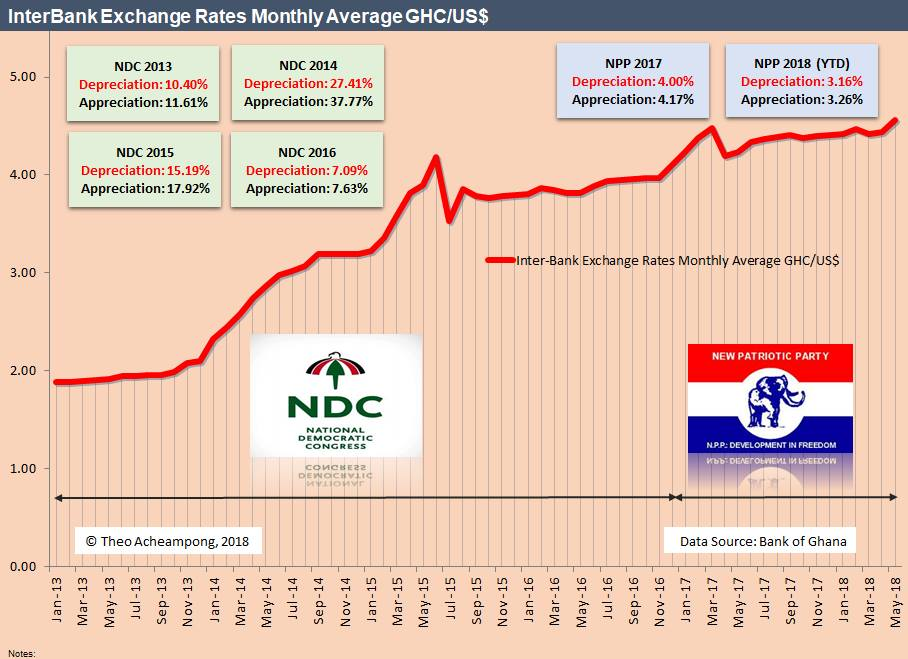# Cedi appreciation or depreciation? The conceptual framework for calculating depreciation and appreciation of currencies

On 21 June 2014, amid the heavy debate on cedi depreciation and ill-timed Bank of Ghana forex rules, I wrote a piece on this platform explaining the conceptual framework for computing the depreciation or appreciation of currencies. I reproduce it below:

********
Under a floating exchange range regime, depreciation refers to the loss of value of a country’s currency with respect to one or more foreign (reference/basket) currencies often due to a number of reasons – economic fundamentals, interest rate differentials, political instability, risk aversion among investors, etc. Countries with weak economic fundamentals such as chronic current account deficits and high rates of inflation generally have depreciating currencies [Investopedia]. For example, if the Ghana Cedi depreciates relative to the dollar within a given timeframe, the exchange rate, which is the Ghana Cedi price of dollars, rises. That is, it takes more Ghana Cedis to purchase one dollar.

In the 36 months ending May 2014, for example, the Ghana Cedi depreciated by 49.87% against the US dollar or the dollar appreciated by 99.46% against the Cedi. Question: how is this computed and what is the cause of the confusion surrounding the rate computation? Well, it’s actually to do with the conceptual framework of the upper bound for depreciation and the percent change formula, which many including experts alike, have confused to represent depreciation. This, however, is notwithstanding the real substance of the debate, which is the ill-timed and ineffective Bank of Ghana Forex rules.

The formula below hopefully should clear this confusion:
1. Depreciation of currency = [Old Rate – New Rate] /New Rate x 100%. That always generates a negative number for which the bound cannot be beyond 100% as the denominator is the greater number.
2. Appreciation of currency = [New Rate – Old Rate]/ Old Rate. Hence, we can have greater than 100% appreciation.

The key here is what one chooses as the base currency and quoted currency. Let me explain this further. Using USD/GHC, which is how many Ghana Cedis is a dollar worth; In May 2013, the midpoint USD/GHC was 1.9867 as compared to 2.9052 in May 2014. Hence, the depreciation over the 12-month period for the Cedi compared to the dollar was -31.62% [1.9867-2.9052/2.9052].

Alternatively, the dollar appreciated against the Cedi by 46.23% [2.9052-1.9867/1.9867]. On the other hand, using GHC/USD (how many dollars a cedi is worth) in May 2013, GHC/USD was 0.5034 compared to 0.3443 in May 2014. In this instance, you would use the former formula to compute the depreciation. Which is [0.3443-0.5034]/0.5034 *100% = -31.61% depreciation of the Cedi relative to the dollar or relative to the Cedi the dollar appreciated by 46.21%. That is [0.5034-0.3443]/ 0.3443*100% = 46.21%. Note this is the reverse of the formula above.

As I have demonstrated, the formula is a bit tricky. In the discussions taking place on Social Media, I have noticed many (myself included) sometimes adopt the usual percentage change formula, which is incorrect. Depreciation of a currency cannot be more than 100%; appreciation, on the other hand, can be. Therefore, anything above 100% is appreciation and not depreciation. What most folks have been referring to and/or computing is the latter, which is the appreciation of the dollar relative to the Cedi.

Have a good weekend!